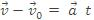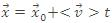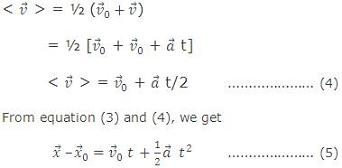Click to Chat

1800-1023-196

+91-120-4616500

CART 0

• 0

MY CART (5)

Use Coupon: CART20 and get 20% off on all online Study Material

ITEM
DETAILS
MRP
DISCOUNT
FINAL PRICE
Total Price: Rs.

There are no items in this cart.
Continue Shopping
        formulas list of ch-motion in straight line?also some importantion questions for annual exams according to CBSE
3 months ago

							 Equations of MotionThe relationship among different parameter like displacement velocity, acceleration can be derived using the concept of average acceleration and concept of average acceleration and instantaneous acceleration.When acceleration is constant, a distinction between average acceleration and instantaneous acceleration loses its meaning, so we can writewhere  is the velocity at t = 0 and  is the velocity at some time t.So,.................. (2)This is the first useful equation of motion.Similarly for displacement.................. (3)in which  is the position of the particle at t0 and  is the average velocity between t0 and later time t. If at t0 and t the velocity of particle isThis is the second important equation of motion.Now from equation (2), square both side of this equation we getThis is another important equation of motion.

3 months ago
Think You Can Provide A Better Answer ?

## Other Related Questions on General Physics

View all Questions »### Course Features

• 731 Video Lectures
• Revision Notes
• Previous Year Papers
• Mind Map
• Study Planner
• NCERT Solutions
• Discussion Forum
• Test paper with Video Solution### Course Features

• 18 Video Lectures
• Revision Notes
• Test paper with Video Solution
• Mind Map
• Study Planner
• NCERT Solutions
• Discussion Forum
• Previous Year Exam Questions